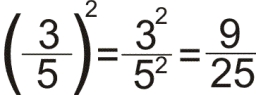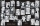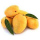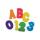# Factors 2

A number has 3 identical factors. If 1 of them is 18. Find the number step by step

x =  5832

### Step-by-step explanation:Did you find an error or inaccuracy? Feel free to write us. Thank you!## Related math problems and questions:

• How many 15How many six-eights are there in 18? Which of the following would you choose to solve this question? 18 x 6/8 or 18 : 6/8
• Summer campSome boys or girls signed up for the summer camp, which has a maximum capacity of 200 children. The main leader noticed that during the evening start, he could arrange the participants exactly in the twelve-step, sixteen-step, or eighteen-step, and no one
• Prime factorsFactor the number 6600 into the product of prime numbers.
• DigitsHow many odd four-digit numbers can we create from digits: 0, 3, 5, 6, 7? (a) the figures may be repeated (b) the digits may not be repeated
• Prime factorsWrite 98 as a product of prime factors
• Simplify 7Simplify. 7-1/3÷ 3-2/3 of 2+ 4-1/2÷ 2-1/4+ 1/2 solution and by step by step
• Sum of fractionsWhat is the sum of 2/3+3/5?
• Identical cubesFrom the smallest number of identical cubes whose edge length is expressed by a natural number, can we build a block with dimensions 12dm x 16dm x 20dm?
• Closest natural numberFind the closest natural number to 4.456 to 44.56 and 445.6.
• MangoesChris has 12 mangoes and Jay has 18 mangoes. Each of them will share the mangoes to their friends . What's the greatest number of mangoes each of their friends get if Chris and Jay will give the same number of mangoes?
• A number 5A number is divisible by 24, 25, and 120 if it is increased by 20. Find the number.
• Find unknown numberWhat is the number between 50 and 55 that is divisible by 2,3,6,9?
• Unknown numberSamuel wrote unknown number. Then he had add 200000 to the number and the result multiply by three. When it calculated he was surprised, because the result would have received anyway, if write digit to the end of original number. Find unknown number.
• Find the meanFind the number between 13 and 29.
• Taken away from number18 is taken away from 8 times of a number is 30. Find the number.
• The buildingThere are 45 people living in building, out of these 3/5 are men,2/5 are women . find the number of men and women in the building.
• Divisibility by 12Replace the letters A and B by digits so that the resulting number x is divisible by twelve /find all options/. x = 2A3B How many are the overall solutions?